# NCERT Solutions For Class 9 Math Chapter – 7 Exercise – 7.3

NCERT Solutions For Class 9 Math Chapter – 7 Exercise  7.3

Q1. ABC and DBC are two isosceles triangles on the same base  BC and vertices A and D are on the same side of BC If AD is extended to intersect BC at P, Show that

• ABD ∆ACD
• ABP ACP
• AP bisects ∟A as well as ∟D.
• AP is the perpendicular bisector of BC.

Solution:-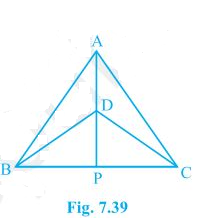• In triangle ABD and ACD.

AB = AC

BD = CD

∆ABD  ∆ACD [SSS Rule]

• In triangle ABP and ACP

AB = AC

AP = PA

∟BAP

= ∟CAP

∆ABP  ∆ACP

BP = PC ∟BPA = ∟CPA

• ∟BAP = ∟CAP

AP bisects ∟A.

∟BDP = ∟CDP

So , DP bisects ∟D.

AP bisects ∟A as well as ∟D.

• ∟BPA + ∟CPA = 1800

2∟BPA = 1800

∟BPA = 900

AP bisects BC.

Q2. AD is an altitude of an isosceles  triangle ABC  in which AB = AC Show that

Solution:-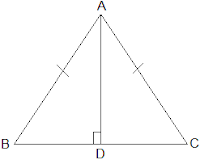∆ABD  ∆ACD [RHS Rule]

• BD = CD

Q3. Two sides AB and BC and median AM of one triangle ABC are respectively equal to sides PQ and QR and median PN of ∆PQR Show that:

• ABM PQN
• ABC

Solution:-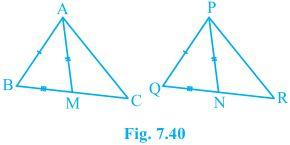• M and N are mid – points of BC and QR respectively, As AM and PN are medians.

BM = 1/2BC and QN = 1/2QR

Also , BC = QR

1/2BC = 1/2QR = BM = QN

Consider triangles ABM and PQN,

AB = PQ

AM = PN

BM = QN

∆ABM  ∆PQN [SSS Rule]

• From Result (i)

∟ABM = ∟PQN

∟ABC = ∟PQR  (iii)

AB = PQ

BC = QR

∟ABC = ∟PQR

∆ABC  ∆PQR. [SAS rule]

Q4. BE and CF are two equals altitude of a triangle ABC Using RHS Congruence rule , Prove that  the triangle ABC is isosceles.

Solution:-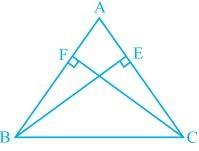∆BFC  ∆CEB [RHS Rule]

∟FBC = ∟ECB

∟ABC = ∟ACB

AC  = AB

[ Sides opposite to equal angels of a triangle are equal.]

∆ABC is an isosceles triangle.

Q5. ABC is an isosceles triangle with AB = AC Draw AP perpendicular BC to show that ∟B = ∟C.

Solution:-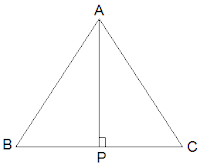AB = BC

AP = PA

∟APB =  ∟APC

∆APB  ∆ACP

∟B = ∟C.